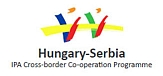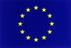## Visualized problems in the teaching topic "derivative of a function"

Kulcsszavak: Geogebra, calculus, derivative, visualization, motion problems
Absztrakt:

Some possibilities for implementing cognitive-visual approach in calculus are visualized problems. These are problems in which the image is explicitly or implicitly included in the very manner the problem is formulated, in the way of solving the problem or in the final solution. We are presenting examples of how GeoGebra is used in classrooms, to explain and explore concept of first derivative and its properties and applications. The concept of local extreme values of functions is also one of the themes in this teaching material. The basic idea while preparing GeoGebra dynamic worksheets is connecting algebraic and geometrical interpretation of the concept of derivative of a function.

Uniform motion and motion problems are teaching topics which are taught in physics class in  school. The problems of uniform movement are an integral part of the teaching contents of mathematics, both in primary as well as secondary school. Mathematical and physical approach to the solution of specific tasks may vary. In the second part of teaching materials we present how GeoGebra can be used in modeling the problem of uniform movement and movement problems and how the created models can be applied in the classroom. We prepared two simulations, one which refers to uniform motion and the other refers to motion problems realised with application of derivations and integrals.

The development is co-financed by the European Union in the frame of the project IPA HU-SRB/1203/221/024: Non-Standard Forms of Teaching Mathematics and PhysicsSzükséges szoftverek:

Geogebra

Tartalomjegyzék:

Click to the document